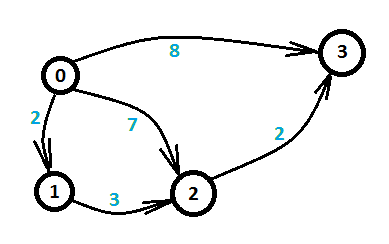# 浅谈差分约束问题

## 思路

$x[j]-x[i]<=k$

$x[j]<=x[i]+k$

$d[v]<=d[u]+k$（k表示边权）1.(3)                 x3 - x0 <= 8
2.(2) + (5)           x3 - x0 <= 9
3.(1) + (4) + (5)     x3 - x0 <= 7## 例题

POJ1201

POJ1275

HDU3440

posted @ 2018-03-06 18:04  自为风月马前卒  阅读(...)  评论(... 编辑 收藏

……